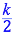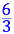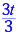## OBJECTIVE: To learn about the axioms of equality

In any sport, there are basic rules by which the game is played. The same is true for math. The basic rules of algebra are called AXIOMS or PROPERTIES. In this lesson, you'll learn about the AXIOMS OF EQUALITY. If a, b, and c are elements of the set of real numbers, the following AXIOMS OF EQUALITY apply: 1. REFLEXIVE AXIOM — a = a 2. SYMMETRIC PROPERTY — If a = b, then b = a. 3. TRANSITIVE PROPERTY — If a = b and b = c, then a = c. 4. SUBSTITUTION PROPERTY — If a = b, then a may be substituted with b wherever it occurs. 5. ADDITION PROPERTY — If a = b, then a + c = b + c. 6. SUBTRACTION PROPERTY — If a = b, then a - c = b - c. 7. MULTIPLICATION PROPERTY — If a = b, then a • c = b • c. 8. DIVISION PROPERTY — If a = b, then a ÷ c = b ÷ c. Algebraic Examples: 1. REFLEXIVE AXIOM 15 = 15 2. SYMMETRIC PROPERTY — If n = 2, then 2 = n. 3. TRANSITIVE PROPERTY — If y = 32 and 32 = 9, then y = 9. 4. SUBSTITUTION PROPERTY — If x = 7, then 2x = 2(7). 5. ADDITION PROPERTY — If x = -4, then x + 4 = -4 + 4. 6. SUBTRACTION PROPERTY — If r + 1 = 7, then r + 1 - 1 = 7 - 1. 7. MULTIPLICATION PROPERTY — If= 8, then(2) = 8(2). 8. DIVISION PROPERTY — If 6 = 3t, then=.
Below is a set of problems involving axioms of equality.

## In this lesson, you learned about the axioms of equality.

In future lessons, you'll learn other axioms pertaining to real numbers. Hope you ENJOYED this lesson!